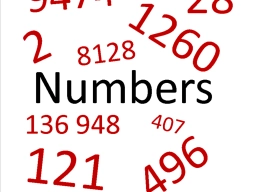# Expression values

Let A = 5, B = 4.4, and C = 4.25. Find the value of each expression listed below.

A2 × (B - C)
B × (A - C)
B + C - A
A - B + C

a =  3.75
b =  3.3
c =  3.65
d =  4.85

### Step-by-step explanation:Did you find an error or inaccuracy? Feel free to write us. Thank you!